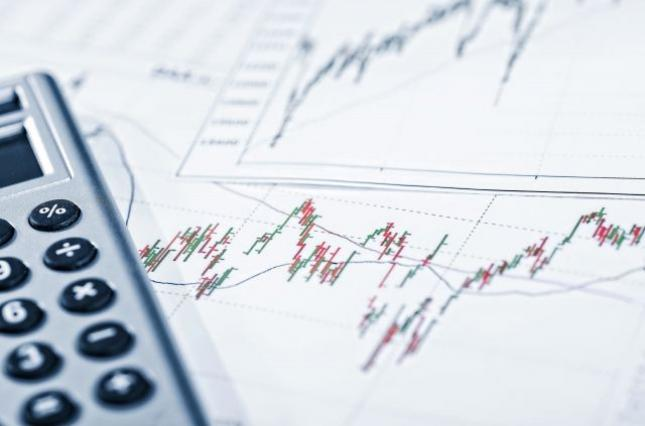20:43 / 01.05.2021
3103
Statistics Committee announces inflation rate for AprilIn April 2021, goods and services in the consumer market rose in price by an average of 1.5%, follows from the State Statistics Committee data.

Since the beginning of this year, the price increase was 4.1%, with an average monthly rate of 1.0%. The annual consumer price index (CPI) grew by 10.7%.

In April 2020, goods and services rose by an average of 1.7% during the month. In January-April 2020, the overall CPI growth rate was 4.5% and the average monthly growth rate was 1.1%. In April 2020, the annual total CPI reached 14.5%.

In April 2021, the short-term overall CPI was lower by 0.2 percentage points than in April of the previous year and higher by 0.6 percentage points than in April 2019.

The total CPI at the annual rate in April 2021 (compared to the corresponding month of the previous year) was lower by 3.8 percentage points than in April 2020 and lower by 3.0 percentage points than in April 2019. The figure for January-April 2021 (compared to December last year) was lower by 0.4 percentage points than the corresponding figure for January-April last year and lower by 1.2 percentage points than the corresponding figure for 2019.

In January-April 2021, food prices rose by 6.2%, lower by 1.5 percentage points than in the corresponding period of 2020 and lower by 1.2 percentage points than the corresponding figure in 2019.

Compared to December 2020, non-food prices rose by 2.1%, which is lower by 0.3 percentage points than the corresponding figure for the previous year and lower by 1.8 percentage points than the corresponding figure for 2019.

Related News
Top